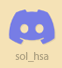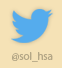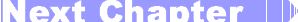Sol::Tutorials

# Sol's Graphics for Beginners(ch07.cpp)

## (Last revised 9. August 2005)

Several people have noted that the logical extension to the graphics tutorial would be a game. The part B of this tutorial consists of only one application; a ball game. As such it's somewhat different from part A. Since we're building a game, we'll be going through several issues that are important in a game application.

The goal is to make a game in which you control a ball in a labyrinth, collecting some gems (or some other valuables), and finally navigate to the goal. The labyrinth is a floating platform hovering over a bottomless pit; if the player doesn't stay on the platform, the game is over.

We'll be controlling the ball with either a joystick or the cursor keys.

You can download the finished game binaries (win32) if you want to see the end result.

Building a game like this is a very iterative process. Some of that nature can be seen in this step-by-step tutorial. First we write a simple implementation, which gets replaced by a more complex one, which gets modified later on, etc. Most of the iterations I went through are not shown here (such as when I was playing around with collision detection and ended up discarding 50 lines of code in favor of one line).

First off, let's start with a simple skeleton, which is pretty close to what you have seen so far in the earlier chapters. As such, this chapter is mostly a source listing; there's very little new stuff in here.

Make a copy of some earlier chapter, and copy-paste the following over the whole source file (or, download the source (right-click, save as)):

``````
#include <stdlib.h>
#include <string.h>
#include <math.h>
#if defined(_MSC_VER)
#include "SDL.h"
#else
#include "SDL/SDL.h"
#endif

// Screen surface
SDL_Surface *gScreen;

// Screen pitch
#define PITCH (gScreen->pitch / 4)
// Screen width
#define WIDTH 480
// Screen height
#define HEIGHT 320
// Color of player's ball
#define BALLCOLOR 0x007fff
// Radius of the player's ball
// Background color
#define BGCOLOR 0x5f0000
// Wall color
#define WALLCOLOR 0x9f1f1f
// Definition of PI
#define PI 3.1415926535897932384626433832795f

// Player's position
float gXPos;
float gYPos;

void drawcircle(int x, int y, int r, int c)
{
int i, j;
for (i = 0; i < 2 * r; i++)
{
// vertical clipping: (top and bottom)
if ((y - r + i) >= 0 && (y - r + i) < HEIGHT)
{
int len = (int)sqrt((float)(r * r - (r - i) * (r - i))) * 2;
int xofs = x - len / 2;

// left border
if (xofs < 0)
{
len += xofs;
xofs = 0;
}

// right border
if (xofs + len >= WIDTH)
{
len -= (xofs + len) - WIDTH;
}
int ofs = (y - r + i) * PITCH + xofs;

// note that len may be 0 at this point,
// and no pixels get drawn!
for (j = 0; j < len; j++)
((unsigned int*)gScreen->pixels)[ofs + j] = c;
}
}
}

void drawrect(int x, int y, int width, int height, int c)
{
int i, j;
for (i = 0; i < height; i++)
{
// vertical clipping: (top and bottom)
if ((y + i) >= 0 && (y + i) < HEIGHT)
{
int len = width;
int xofs = x;

// left border
if (xofs < 0)
{
len += xofs;
xofs = 0;
}

// right border
if (xofs + len >= WIDTH)
{
len -= (xofs + len) - WIDTH;
}
int ofs = (i + y) * PITCH + xofs;

// note that len may be 0 at this point,
// and no pixels get drawn!
for (j = 0; j < len; j++)
((unsigned int*)gScreen->pixels)[ofs + j] = c;
}
}
}

void init()
{
gXPos = WIDTH / 2;
gYPos = HEIGHT / 2;
}

void render()
{
// Ask SDL for the time in milliseconds
int tick = SDL_GetTicks();

// Lock surface if needed
if (SDL_MUSTLOCK(gScreen))
if (SDL_LockSurface(gScreen) < 0)
return;

// fill background
drawrect(0, 0, WIDTH, HEIGHT, BGCOLOR);

// draw borders
drawrect(0, 0, WIDTH, 8, WALLCOLOR);
drawrect(0, 0, 8, HEIGHT, WALLCOLOR);
drawrect(WIDTH - 8, 0, 8, HEIGHT, WALLCOLOR);
drawrect(0, HEIGHT - 8, WIDTH, 8, WALLCOLOR);

// draw the player object
drawcircle((int)gXPos,
(int)gYPos,
BALLCOLOR);

// Unlock if needed
if (SDL_MUSTLOCK(gScreen))
SDL_UnlockSurface(gScreen);

// Tell SDL to update the whole gScreen
SDL_UpdateRect(gScreen, 0, 0, WIDTH, HEIGHT);
}

// Entry point
int main(int argc, char *argv[])
{
// Initialize SDL's subsystems - in this case, only video.
if (SDL_Init(SDL_INIT_VIDEO) < 0)
{
fprintf(stderr, "Unable to init SDL: %s\n", SDL_GetError());
exit(1);
}

// Register SDL_Quit to be called at exit; makes sure things are
// cleaned up when we quit.
atexit(SDL_Quit);

// Attempt to create a WIDTHxHEIGHT window with 32bit pixels.
gScreen = SDL_SetVideoMode(WIDTH, HEIGHT, 32, SDL_SWSURFACE);

init();

// If we fail, return error.
if (gScreen == NULL)
{
fprintf(stderr, "Unable to set up video: %s\n", SDL_GetError());
exit(1);
}

// Main loop: loop forever.
while (1)
{
// Render stuff
render();

// Poll for events, and handle the ones we care about.
SDL_Event event;
while (SDL_PollEvent(&event))
{
switch (event.type)
{
case SDL_KEYDOWN:
break;
case SDL_KEYUP:
// If escape is pressed, return (and thus, quit)
if (event.key.keysym.sym == SDLK_ESCAPE)
return 0;
break;
case SDL_QUIT:
return(0);
}
}
}
return 0;
}
``````

The first new bit is a block of #define lines. Here we're defining a bunch of constants (most of these could be - and, it could be argued that they should be - 'const' variables).

After the #defines we have two global variables - gXPos and gYPos. These will contain the coordinates of the player's object.

The drawcircle() function should be familiar from Primitives and Clipping. Below the circle drawing is a simple rectangle drawing, which is very similar to the circle drawing function. The main differences being separate width and height, and that each scanline has the same width.

In init(), we're setting the player's position to the center of the screen. Oh, notice that we're using the WIDTH and HEIGHT macros instead of simply saying 640 and 480 here. In fact, the WIDTH and HEIGHT are 480 and 320 for this example. If you wanted, you could change the resolution easily by changing the values of WIDTH and HEIGHT. (Feel free to play with them, but change them back when you're done).

The render() function clears out the display with a call to drawrect(), and then draws some borders using four drawrect():s. The code has been written using the #defined constants so that you can change the display resolution and the result will still be the same. Finally, the player object is drawn using drawcircle().

The main() has also been changed to use the constants instead of hardcoded values.

Now, if you compile and run, you'll see a static screen which is the result of the render() function. Our first step to turn this into a game is to introduce 08 - Some Interactivity.Having problems? Improvement ideas? Just want to discuss this tutorial? Try the forums!

Any comments etc. can be emailed to me.